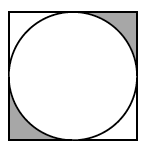### Home > INT2 > Chapter 9 > Lesson 9.4.1 > Problem9-137

9-137.The diagram at right shows a circle inscribed in a square. What is the area of the shaded region if the side length of the square is $6$ units?

Find the area of the square. Then subtract the area of the circle to find the areas
of the corners. What number can you divide by in order to then find the shaded region.

$(36 - 9π) ÷ 2 ≈ 3.86$ units$^2$